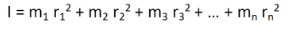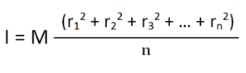Checkout JEE MAINS 2022 Question Paper Analysis : Checkout JEE MAINS 2022 Question Paper Analysis :

The snapshot of the moment of inertia of a body about an axis is in some cases spoke to utilize the radius of gyration. Now, what actually is the radius of gyration? We can characterize the radius of gyration as the fanciful good ways from the centroid at which the territory of the cross-area is envisioned to be engaged at a point to get a similar moment of inertia.

## What is Radius of Gyration?

We can define the radius of gyration as the imaginary distance from the centroid at which the area of cross-section is imagined to be focused at a point in order to obtain the same moment of inertia. It is denoted by k.

The formula of moment inertia in terms of the radius of gyration is given as follows:

I = mk2       (1)

where I is the moment of inertia and m is the mass of the body

Accordingly, the radius of gyration is given as follows

$$\begin{array}{l}k=\sqrt{\frac{I}{m}}\end{array}$$
(2)

The unit of the radius of gyration is mm. By knowing the radius of gyration, one can find the moment of inertia of any complex body equation (1)  without any hassle.

Consider a body having an n number of particles each having a mass of m. Let the perpendicular distance from the axis of rotation be given by r1, r2, r3,…,rn. We know that the moment of inertia in terms of radius of gyration is given by the equation (1). Substituting the values in the equation, we get the moment of inertia of the body as follows………… (3)

If all the particles have the same mass then equation (3) becomesWe can write mn as M which signifies the total mass of the body. Now the equation becomes………… (4)

From equation (4), we getFrom the above equation, we can infer that the radius of gyration can also be defined as the root-mean-square distance of various particles of the body from the axis of rotation.

### What is the use of the radius of gyration?

The radius of gyration is used to compare how various structural shapes will behave under compression along an axis. It is used to predict buckling in a compression beam or member.

## Frequently Asked Questions – FAQs

Radius of Gyration is defined as the imaginary distance from the centroid at which the area of cross-section is imagined to be focused at a point in order to obtain the same moment of inertia.

### How is the Radius of Gyration denoted?

Radius of Gyration is denoted by k.

### What is the formula of Radius of Gyration?

Radius of Gyration is given as follows:
$$\begin{array}{l}k=\sqrt{\frac{I}{m}}\end{array}$$
Where I is the moment of inertia and m is the mass of the body.

### What are the uses of Radius of Gyration?

The radius of gyration is used to compare how various structural shapes will behave under compression along an axis. It is used to predict buckling in a compression beam or member.

### What is the unit of Radius of Gyration?

The unit of the Radius of Gyration is mm.Stay tuned to BYJU’S to know more about uniform circular motion along with other topics in physics like gravitation, laws of motion, and more with the help of interactive video lessons.